• 選課中心
• 手機版掃描進入手機版

• 官方APP掃描或下載APP

• 微信服務掃描關注微信

• 官方微博掃描關注微博

# SAT2物理公式總結

2017-07-17 17:53:26 來源：新東方在線

新東方在線SAT頻道為大家帶來SAT2物理公式總結一文，希望對大家SAT備考有所幫助。更多精彩盡請關注新東方在線SAT頻道!

SAT2物理熱力學常見公式：

Heat added or emoved: Q = m·c·ΔT

Change in Internal Energy: ΔU = Q - W

Boyle's Law: P1V1 = P2V2

Charles's Law: V1/T1 = V2/T2

Efficiency of a heat engine(%): E = (W/Qhot)·100

SAT2物理光波學常見公式：

Snell's Law: n1·sin(θ1) = n2·sin(θ2)

Wave speed: v = λ·f

Velocity of light: v = c/n

Magnification: m = -di/do

Law of Reflection: θreflection = θincidence

Mirror and lens equation: 1/d1 + 1/d1 = 1/f

SAT2物理電磁學常見公式：

Electric Fields and Forces Formulas

Coulomb's Law: F = k·q1·q1/r2

Electric field of a charge q: E = k·q/r2

Work done by electric field: W = q·E·d

Electric field between two metallic plates: E = V/d

DC Circuits Formulas

Ohm's Law: V = I·R

Power dissipated in a resistor: P = I·V = V2/R = R·I2

Resistance: R = ρ·l/A

Equivalent resistance of series resistors: Rs = R1 + R2 +...

Equivalent resistance of parallel resistors: 1/Rp = 1/R1 + 1/R2 +...

Equivalent capacitance of series capacitors: 1/Cs = 1/C1 + 1/C2 +...

Equivalent capacitance of parallel capacitors: Cp = C1 + C2 +...

Magnetic Fields and Forces Formulas

Magnetic force on a moving charge: F = q(v x B) = q·v·B·sin(θ)

Magnetic force on a current carrying wire: F = I·l·B·sin(θ)

Magnetic field created by a current: B = (μo/2π)·(I/r)

[page]

SAT2物理常見公式

1. Physics Formulas – Mechanics

Kinematics Formulas

The Kinematic Equations apply to one-dimensional motion with constant acceleration from point 1, situated d1 from a point of reference to point 2, situated d2 from the same point of reference. v1 is the velocity at point 1 and v2 is the velocity at point 2.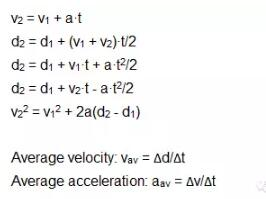Rotational kinematics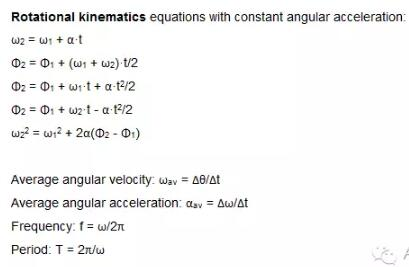Relations between angular and linear variables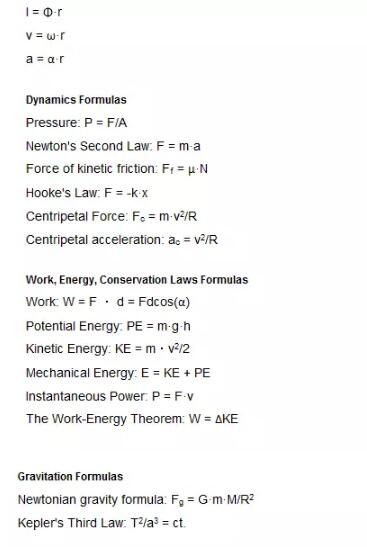2. Thermodynamics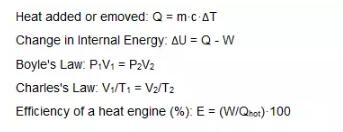3. Waves and Optics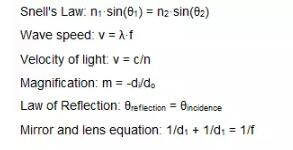4. Modern Physics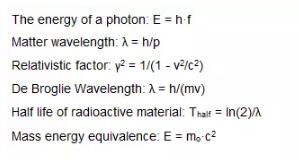5. Electricity and Magnetism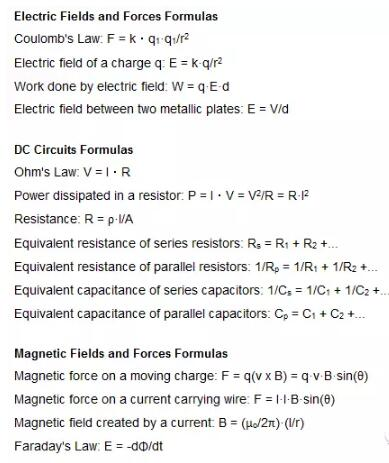SAT2物理力學公式大全

Physics Formulas - Mechanics

Kinematics Formulas

The Kinematic Equations apply to one-dimmensional motion with costant acceleration from point 1, situated d1 from a point of reference to point 2, situated d2 from the same point of reference. v1 is the velocity at point 1 and v2 is the velocity at point 2.

v2 = v1 + a·t

d2 = d1 + (v1 + v2)·t/2

d2 = d1 + v1·t + a·t2/2

d2 = d1 + v2·t - a·t2/2

v22 = v12 + 2a(d2 - d1)

Average velocity: vav = Δd/Δt

Average acceleration: aav = Δv/Δt

Rotational kinematics equations with constant angular acceleration:

ω2 = ω1 + α·t

Φ2 = Φ1 + (ω1 + ω2)·t/2

Φ2 = Φ1 + ω1·t + α·t2/2

Φ2 = Φ1 + ω2·t - α·t2/2

ω22 = ω12 + 2α(Φ2 - Φ1)

Average angular velocity: ωav = Δθ/Δt

Average angular acceleration: αav = Δω/Δt

Frequency: f = ω/2π

Period: T = 2π/ω

Relations between angular and linear variables:

l = Φ·r

v = ω·r

a = α·r

Dynamics Formulas

Pressure: P = F/A

Newton's Second Law: F = m·a

Force of kinetic friction: Ff = μ·N

Hooke's Law: F = -k·x

Centripetal Force: Fc = m·v2/R

Centripetal acceleration: ac = v2/R

Work, Energy, Conservation Laws Formulas

Work: W = F · d = Fdcos(α)

Potential Energy: PE = m·g·h

Kinetic Energy: KE = m·v2/2

Mechanical Energy: E = KE + PE

Instantaneous Power: P = F·v

The Work-Energy Theorem: W = ΔKE

Gravitation Formulas

Newtonian gravity formula: Fg = G·m·M/R2

Kepler's Third Law: T2/a3 = ct.

[page]

SAT2物理黃金公式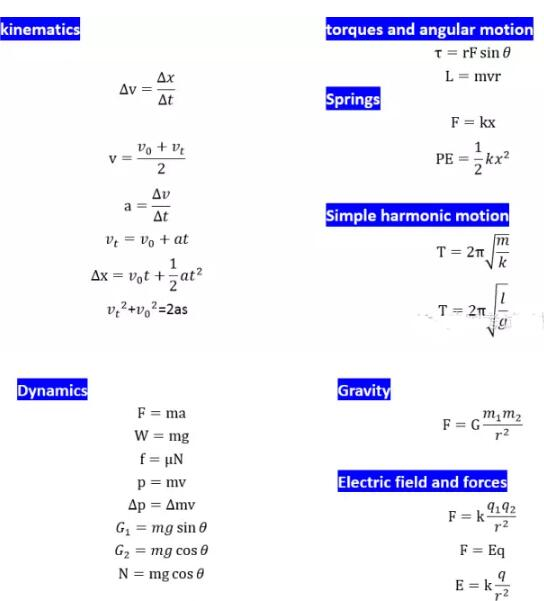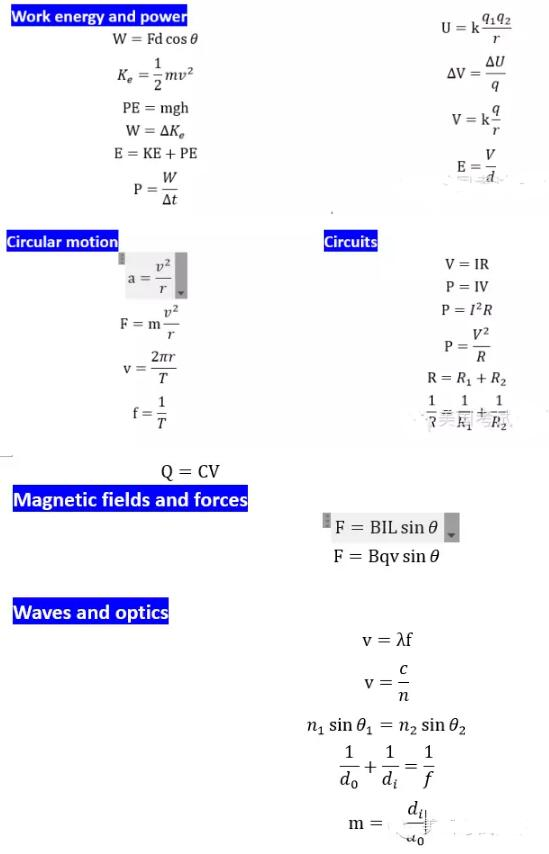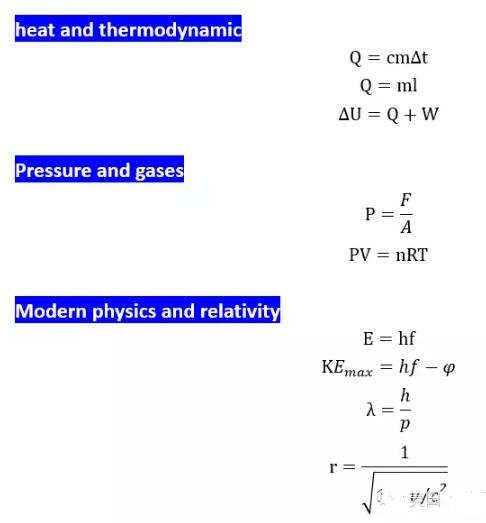以上就是新東方在線SAT頻道為你帶來的SAT2物理公式總結，更多精彩敬請關注新東方在線SAT頻道。

 試聽 試聽

### 四級公開課更多>>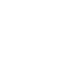w 22分01秒
1 全國大學英語四六級考試聽力重大調整解析
2 視頻：新東方在線2015年12月四級考試綜合點評
3 視頻：新東方在線帥健翔2015年12月四級閱讀真題解析
4 視頻:2015年12月英語四級寫作真題解析
5 2015年12月大學英語四級考試模考卷二